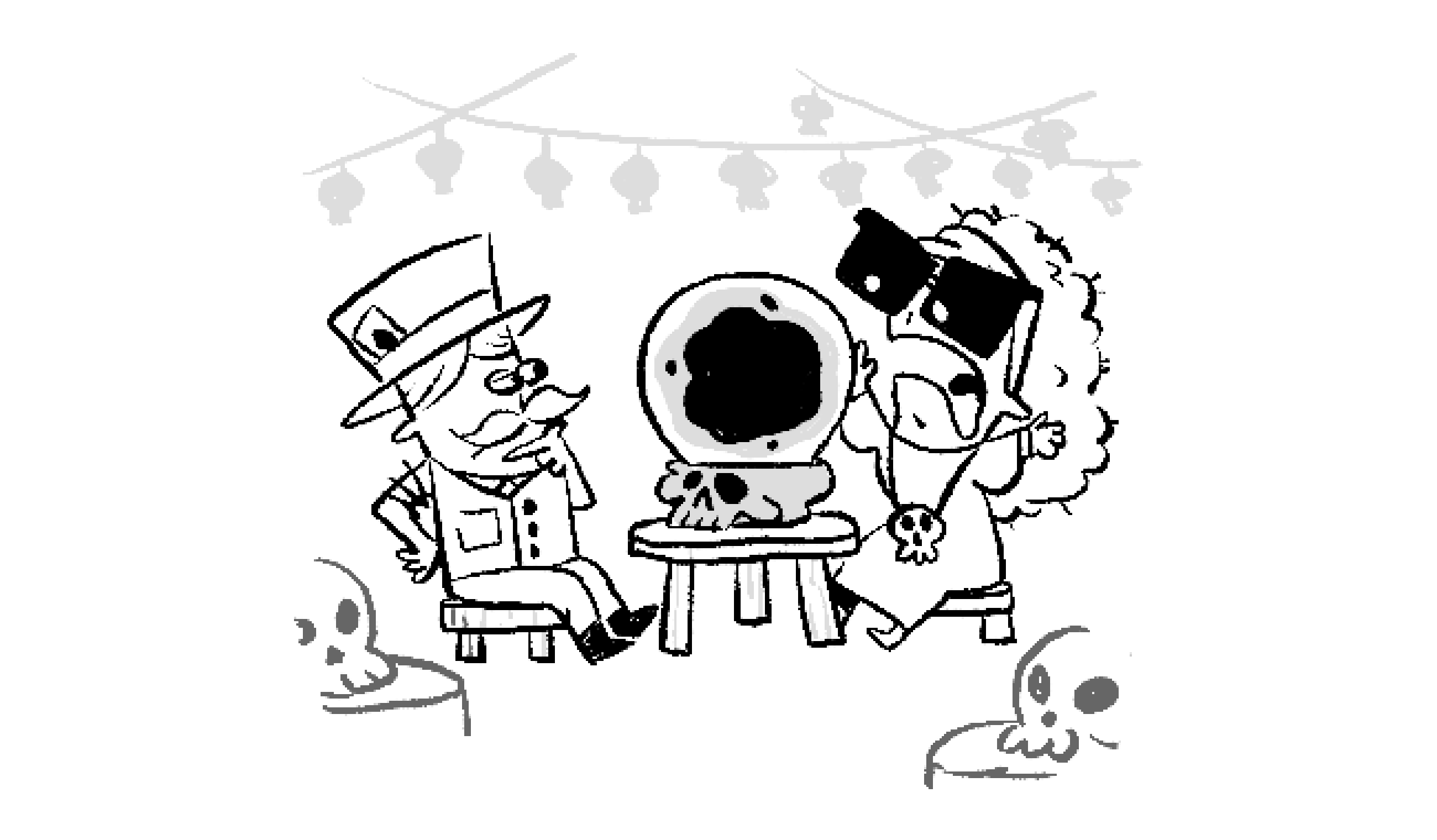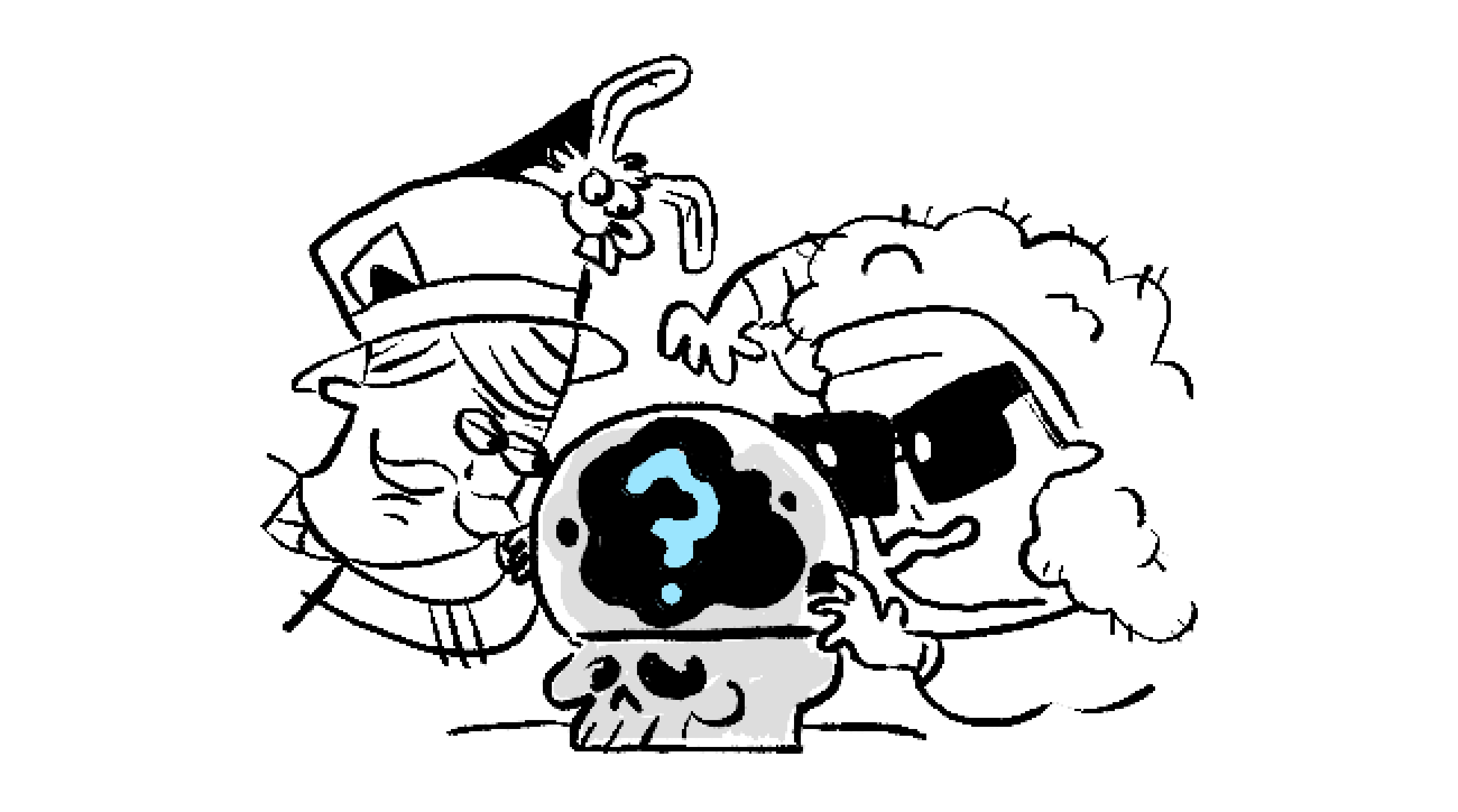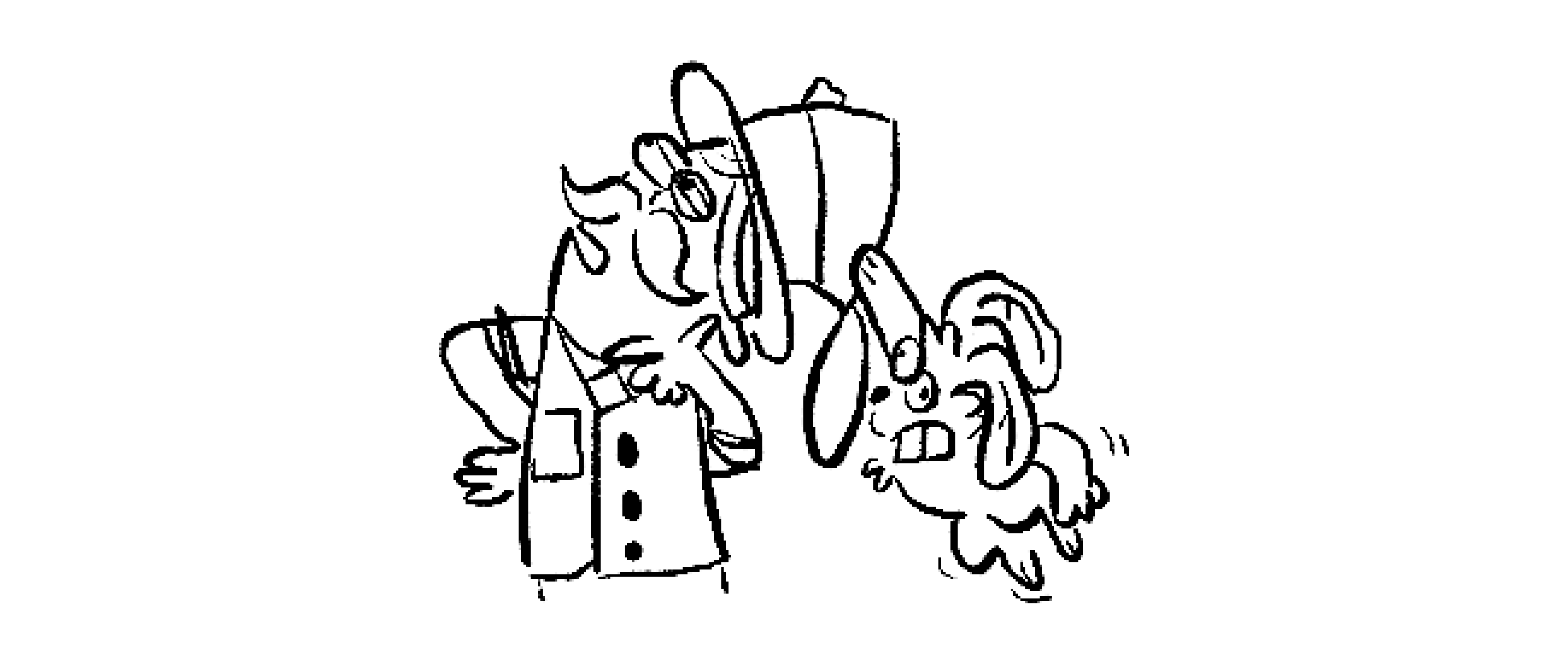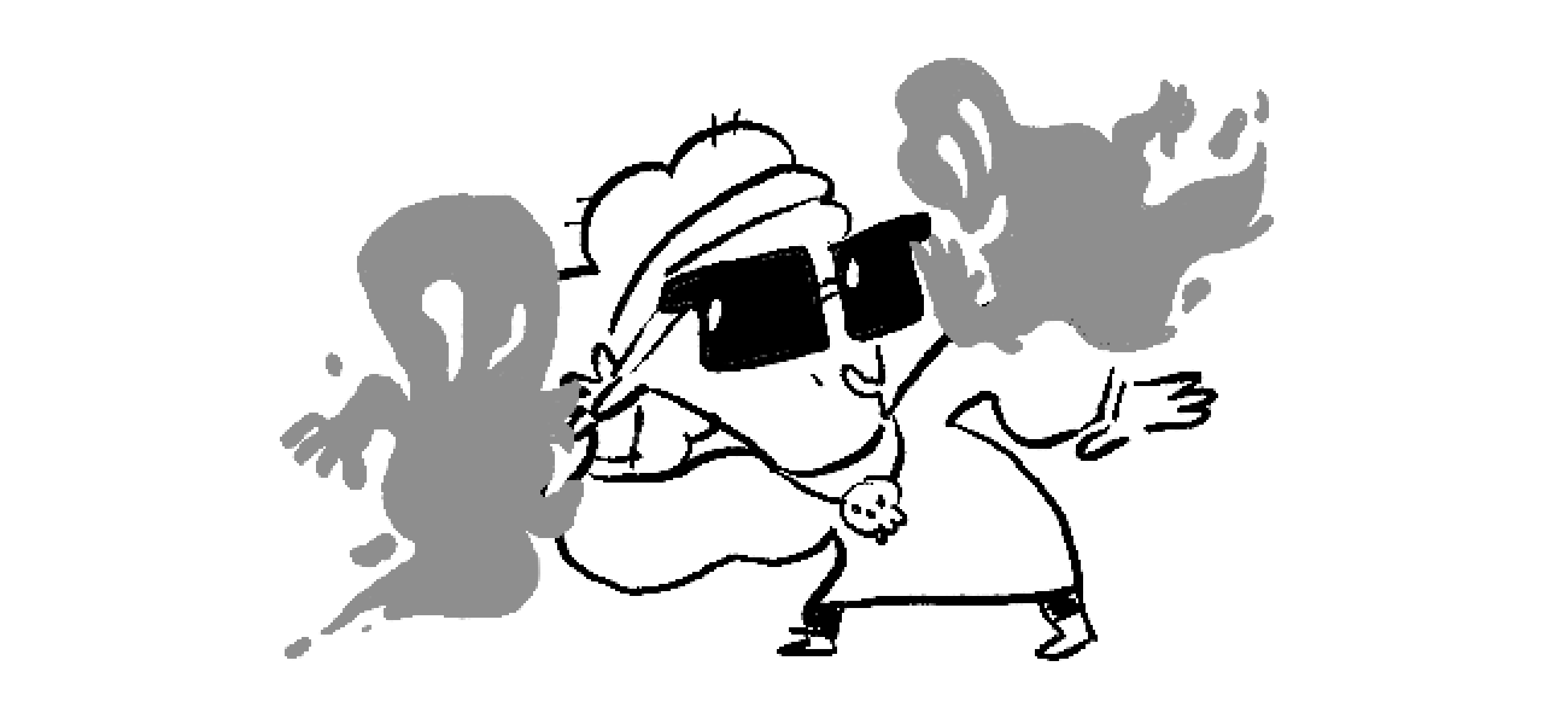### Statistics I

Many of the decisions and judgments we make are based on limited or incomplete information.

Amidst all of this uncertainty, statistics plays a special role:

Statistics provides the tools to make the best possible judgments with limited data.

We have a long way to go before we can fully appreciate how statistics accomplishes this, but this chapter starts us on our journey by introducing the most essential ideas underlying statistics.

In this first quiz, we'll explore how probability is used in statistics to make informed decisions.

# Into the Mystic: Hypothesis Testing

Despite being a magician by trade, Rupert is a skeptic.

Sure, he's built a lucrative career convincing his audience that he can defy the very laws of nature, but it's all sleight-of-hand and well-crafted illusions designed to fool the senses.

So when he hears about Alberta The Oracle, who claims that she draws the answer to any yes or no question from supernatural sources, he sets out to determine the truth for himself.

Rupert thinks Alberta is just a good guesser, so he gathers a stockpile of yes/no questions that only he can answer and then heads out from Magicians Alliance HQ one cold and foggy night to put The Oracle to the test...# Into the Mystic: Hypothesis Testing

In The Oracle's parlor, Rupert takes a seat, thanks his host for the opportunity, and then poses his first question.

Suppose for the moment that Alberta really does just guess the answer to every question she's given by responding "yes" or "no" with equal probability.

What's the probability Alberta answers Rupert's first question correctly just by guessing?# Into the Mystic: Hypothesis Testing

The probabilities in the last problem obey a uniform distribution, which is common in statistics.

To get a sense of what "uniform" means, let's say the set of all possible outcomes of our experiment — called the sample space — is finite.

For example, the sample space in the last problem is $\{ \text{yes}, \text{no} \},$ the only possible responses from The Oracle. Another example is $\{ \tt{HH, TH, HT, TT}\},$ the outcomes for two back-to-back flips of a coin with one side labeled $\tt{H}$ and the other $\tt{T}.$

It's always true that the total probability is $1$ no matter how it's distributed, so if we list the outcomes in the sample space in no particular order, then

\begin{aligned} & \text{(Probability of first outcome)} \\ &+ \text{(Probability of second outcome)} \\ & + \dots + \text{(Probability of last outcome)} \\ &=1. \end{aligned}

In the uniform distribution, all of these probabilities are equal, so

\begin{aligned} & \text{(Probability of an outcome in the uniform distribution)} \\ & \times \text{(Size of the sample space)} = 1, \end{aligned}

or

$\text{(Probability of an outcome in the uniform distribution)} \\[0.8em] = \frac{1}{\text{(Size of the sample space)}}.$

# Into the Mystic: Hypothesis Testing

Suppose Rupert poses three questions to The Oracle.

In that case, her possible responses can be encoded as $3$-letter strings of $\tt{Y}$'s and $\tt{N}$'s.

For example, $\tt{YNY}$ represents Alberta answering "yes" to the first question, "no" to the second, and "yes" to the third. These three-letter strings make up the sample space for Rupert's experiment.

If Alberta simply guesses her answers indiscriminately as Rupert believes, then every outcome in the sample space has the same probability of being observed.

In other words, the probability is distributed uniformly over the sample space.

So what's the probability that Alberta answers Rupert's three questions correctly if the magician is right about the way The Oracle responds?

# Into the Mystic: Hypothesis Testing

To test The Oracle, Rupert needs to know the probability Alberta will answer $n$ consecutive questions correctly if he's right about her method of guessing "yes" and "no" with equal likelihood.

Use what we discovered in the last problem to find this probability.# Into the Mystic: Hypothesis Testing

It's helpful at this point to introduce some terminology that we'll use throughout the course:

A hypothesis is an assumption made before an experiment is done.

Rupert, being a skeptic, hypothesizes that Alberta doesn't in fact commune with otherworldly powers to get her answers: rather, he thinks that she simply guesses "yes" or "no."

# Into the Mystic: Hypothesis Testing

Quizzing The Oracle with his list of questions gives Rupert data, and that data either supports his hypothesis or gives him reason to reject it. But data can never "prove" or "disprove" his hypothesis!

For instance, Alberta could answer correctly for a very long stretch just by random chance, so this doesn't prove she has supernatural knowledge.

On the other hand, she also could receive messages from beyond this world, but her source may not be right all the time: providing one wrong answer doesn't disprove her oracular powers.In short, nothing is ever "proven" in statistics! Hypotheses are either ruled likely true or likely untrue based on data gathered.

# Into the Mystic: Hypothesis Testing

So how exactly does Rupert test his hypothesis that Alberta guesses every answer she gives?

Well, the probability that Alberta gets $n$ consecutive questions correct is $\frac{1}{2^{n}}$ if Rupert's hypothesis is true.

This decays exponentially, so if she did give a long string of correct answers, Rupert's hypothesis looks pretty unlikely: he'd think "Maybe there's something to this oracle business after all!"

Since Rupert is so skeptical, he'll only reject his hypothesis and admit Alberta is an oracle if she gives so many correct answers that the probability she's guessing is less than $0.001.$

How many consecutive correct answers must Alberta give in order for Rupert to change his mind about her abilities?

Use the interactive below if you don't have a calculator handy:

# Into the Mystic: Hypothesis Testing

Rupert's skepticism led him to choose $0.001$ since he needs an "extreme" (i.e. highly unlikely) outcome in order to give up the hypothesis that Alberta simply guesses.

Given what we found in the last problem, Rupert considers $10$ questions answered correctly and consecutively enough to abandon his initial assumption that Alberta guesses her responses.

As we'll see later in the course, there are many practical considerations that go into choosing the threshold at which we give up on a hypothesis, but a probability of $5\%$ is commonly used.

# Into the Mystic: Hypothesis Testing

So what makes Rupert so skeptical of Alberta The Oracle?

Well, truth be told, Rupert often "communes with spirits" in his magic act, too, but the source of his supposed knowledge isn't supernatural: it's all about playing the odds.

Rupert could be drummed out of the Magicians Alliance for revealing his methods, but he's willing to talk to us about one of the simplest tricks that most psychics use:

A quick internet search shows that about $5.5$ million of the $330$ million people living in the US is named James, so a randomly selected American has a probability $p = \frac{1}{60}$ of being a "James."

If a psychic plays to a crowd of size $n$ and says "The spirits tell me there's a James here," how big does $n$ have to be for the psychic to have at least an $80 \%$ chance of being right?

Assume the names of the $n$ audience members are all independent

Hint: Calculate the probability there are no Jameses in the audience, and then use the rule of complement to find the probability there's at least one James. The plot below will be of help.

# Into the Mystic: Hypothesis Testing

Of course, just being $80 \%$ confident there's at least one James in the audience doesn't mean there actually will be one present every time, so a good psychic needs a way to backpedal.

That's why psychics start their acts by explaining that the messages they get can be vague, and then go on to tell the audience that they'll need help understanding what the messages mean.

It's worth taking a moment to think about the probabilistic idea at the center of this magic trick:

No matter how unlikely an event is, the probability of observing it in some experiment goes up with the number of trials you perform.

The probability of randomly selecting a James from the US population is only $1.7 \%,$ but sample $96$ US citizens and the probability one of them is a James is over $80 \%.$

We'll see this idea again later in the course when we look at sources of false positives, which is a type of error made when a hypothesis is rejected even though it's actually true.

# Into the Mystic: Hypothesis Testing

We mentioned this earlier, but it's worth repeating: nothing is ever "proven" with statistics.

Instead, a statistician rejects or doesn't reject a hypothesis based on the "extremeness" of data.

Most statistical analysis boils down to the following steps:

1. $\\[-1em]$Come up with a hypothesis, which is an assumption made before experimentation.
2. Decide on a criterion that rejects the hypothesis if the experimental results are too "extreme."
3. Gather and analyze data, and then reject or don't reject the hypothesis based on the results.$\\[-1em]$

We saw all of these ideas play out in this quiz:

1. $\\[-1em]$First, Rupert hypothesized "The Oracle guesses 'yes' or 'no'."
2. He then came up with a criterion for rejecting it: "If she guesses $10$ correct questions in a row, I think my hypothesis probably isn't true."
3. He gathered data by asking Alberta a series of questions. $\\[-1em]$

Yet this quiz only scratches the surface: our journey to mastering the basic tools and concepts used in modern hypothesis testing truly begins in Chapter $3.$

In the next quiz, we'll introduce two other important aspects of statistics, estimation and sampling, topics we'll take up in full in Chapter $2.$

# Into the Mystic: Hypothesis Testing

×